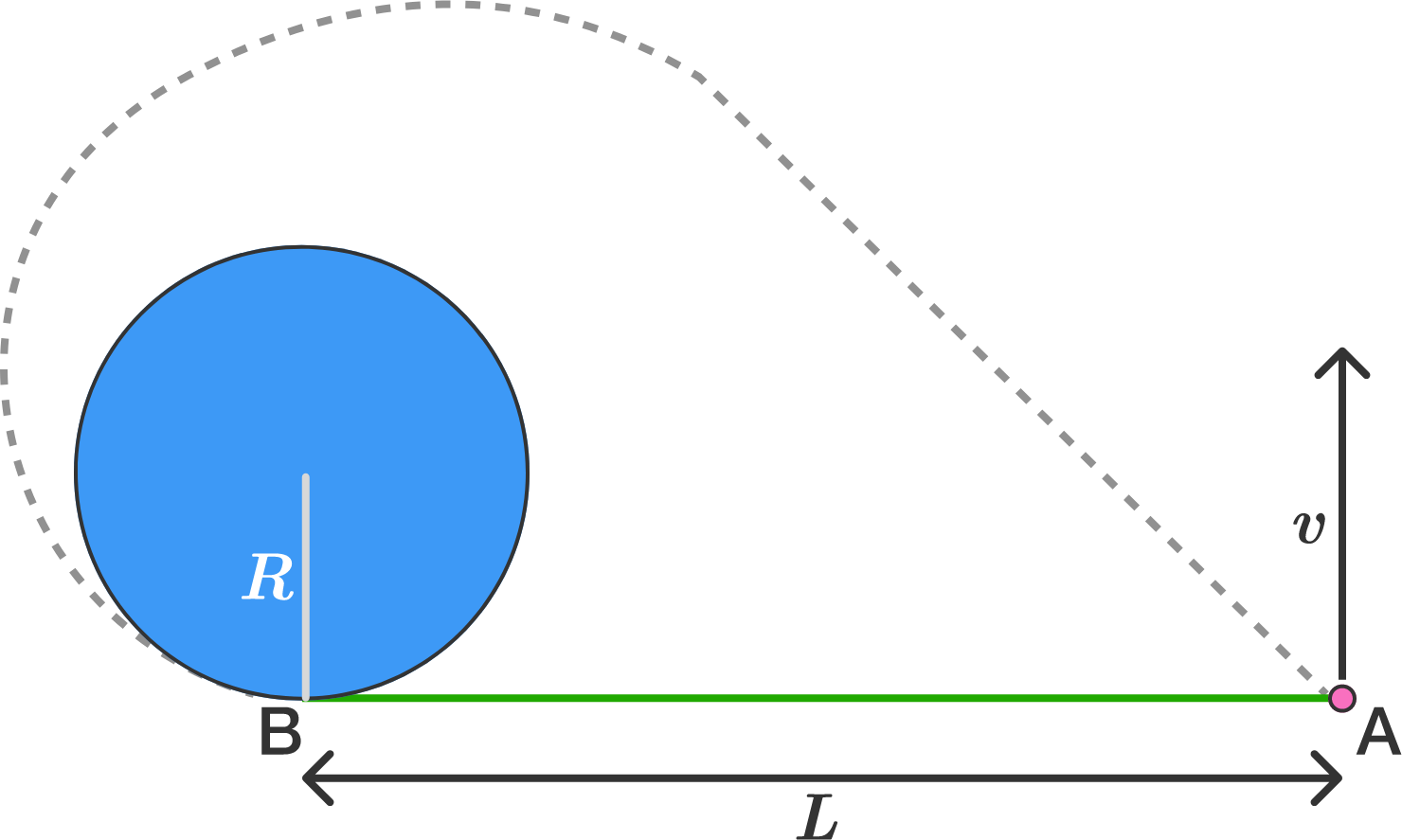A horizontal plane supports a stationary vertical cylinder of radius $R$ and a disc $A$ attached to the cylinder by a horizontal thread $AB$ of length $L$ (see figure above, top view). An initial velocity $v$ is imparted to the disc, as shown above. How long will it move along the plane until it strikes against the cylinder?

Details and Assumptions:

• Neglect friction at all contact surfaces.
• Do not consider gravitational forces.
• The radius of the disc as compared to the radius of the cylinder is very small.
×